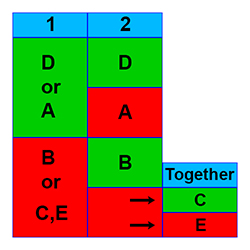# Understanding Data Sufficiency

To see Data Sufficiency Videos, click

Data Sufficiency questions are a unique aspect of the Quantitative section of the GMAT exam. In concept they test your ability to make an evaluation and decision on the feasibility of solving a problem – without actually solving it. This is a typical question for business executives: “Do we have enough information and resources to solve this problem or complete this project?” If you can answer Yes, then you go ahead; otherwise, you have to make some other decision, such as further studying the issue or cancelling the project.

Your task with Data Sufficiency questions is similar. You need to determine whether the data given is sufficient to solve the stated problem. gmatmath.online provides Data Sufficiency practice questions and tests that include solutions and thorough explanations – all of them grouped in nine major areas of mathematics that are tested on the exam.

In the public portion of the site we give you access to over 100 Data Sufficiency questions and question versions with solutions and explanations. Follow this link to view videos that help you understand the Data Sufficiency concept thoroughly. Additionally, in the subscriber-only section you can practice and refine your skills with our bank of thousands of problems and questions.

Every Data Sufficiency (DS) question can have 1 of 5 possible answers, and the possible answers are the same for every DS question. All DS questions are really two questions in one. You’re given a general problem, followed by two supplemental statements. The real problem is to evaluate each of the two statements independently to determine their individual sufficiency to solve the original general problem. As you determine the sufficiency of these statements, you are able to decide which of the following 5 answers to the Data Sufficiency question is the correct answer:

A. Statement 1 is sufficient, but statement 2 is not.

B. Statement 1 is not sufficient, but statement 2 is.

C. Neither statement 1 nor statement 2 is sufficient, but together they are.

D. Both statement 1 and statement 2 are individually sufficient.

E. Neither statement 1 nor statement 2 is sufficient, and together they are not sufficient.The diagram above gives a pictorial representation of the 5 Data Sufficiency answers. The two statement numbers are shown in blue at the top of the first two columns. Note that green means sufficient, and red means not sufficient. As an example, if both statements 1 and 2 are individually sufficient, then the correct answer is D. For more information on the 5 DS answers, see our videos.

You can find over 100 sample Data Sufficiency questions, answers, and explanations on the following pages: